# Statistics - (Residual|Error Term|Prediction error|Deviation) (e| )

The residual is a deviation score measure of prediction error in case of regression.

The difference between an observed target and a predicted target in a regression analysis is known as the residual and is a measure of model accuracy.

The error term is an unobserved variable as:

• it's unsystematic (whereas the bias is)
• we can't see it
• we don't know what it is

In a scatterplot the vertical distance between a dot and the regression line reflects the amount of prediction error (known as the “residual”).

## Equation

### Standard

$e = Y - \hat{Y}$

where in a regression

### Variance and bias

The ingredients of prediction error are actually:

• bias: the bias is how far off on the average the model is from the truth.
• and variance. The variance is how much that the estimate varies around its average.

Bias and variance together gives us prediction error.

This difference can be expressed in term of variance and bias:

$e^2 = var(model) + var(chance) + bias$

where:

• $var(model)$ is the variance due to the training data set selected. (Reducible)
• $var(chance)$ is the variance due to chance (Not reducible)
• bias is the average of all $\hat{Y}$ over all training data set minus the true Y (Reducible)

As the flexibility (order in complexity) of f increases, its variance increases, and its bias decreases. So choosing the flexibility based on average test error amounts to a bias-variance trade-o ff.

Recommended Pages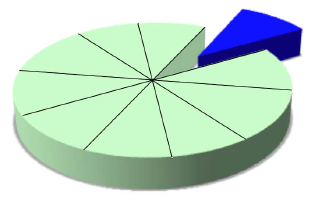(Statistics|Data Mining) - (K-Fold) Cross-validation (rotation estimation)

Cross-validation, sometimes called rotation estimation is a resampling validation technique for assessing how the results of a statistical analysis will generalize to an independent new data set. This...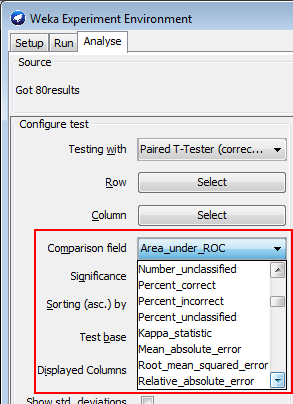Data Mining - (Parameters | Model) (Accuracy | Precision | Fit | Performance) Metrics

Accuracy is a evaluation metrics on how a model perform. rare event detection Hypothesis testing: t-statistic and p-value. The p value and t statistic measure how strong is the...Data Mining - Root mean squared (Error|Deviation) (RMSE|RMSD)

Root mean squared (Error|Deviation) in case of regression. The RMSD represents the sample standard deviation of the differences between predicted values and observed values. The RMSE serves to aggregate...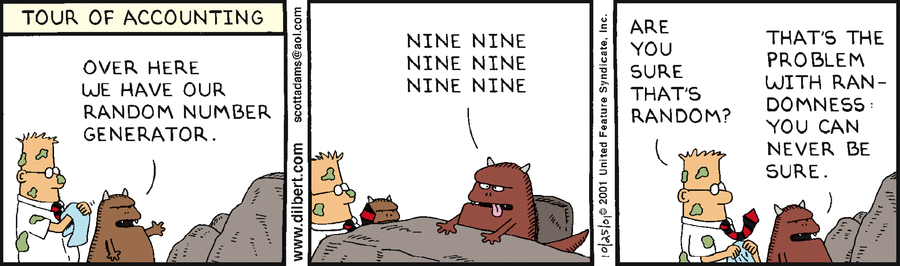Number - Random (Stochastic|Independent) or (Balanced)

Think of randomness as a lack of pattern. Something random should be unpredictable. We shouldn’t be able to predict the next value of the sequence The degree to which a system has no pattern is known...R - Multiple Linear Regression

Multiple linear regression with R functions such as lm Unstandardized Multiple Regression Regression analyses, standardized (in the z scale). The point is a short-cut to select all variables....R - Simple Linear Regression

simple linear regression with R function such as lm Unstandardized Simple Regression Regression analyses, standardized (in the z scale). In simple regression, the standardized regression coefficient...Statistics - (Average|Mean) Squared (MS) prediction error (MSE)

The residual is a measure of prediction error in case of regression based on the residual and is a measure of model accuracy. (Average|Mean) Squared (MS) prediction error (of variance) of Mean Squared...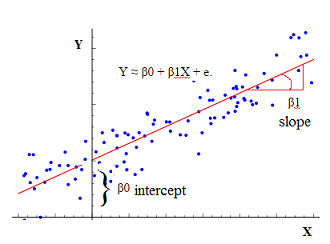Statistics - (Univariate|Simple|Basic) Linear Regression

A Simple Linear regression is a linear regression with only one predictor variable (X). Correlation demonstrates the relationship between two variables whereas a simple regression provides an equation...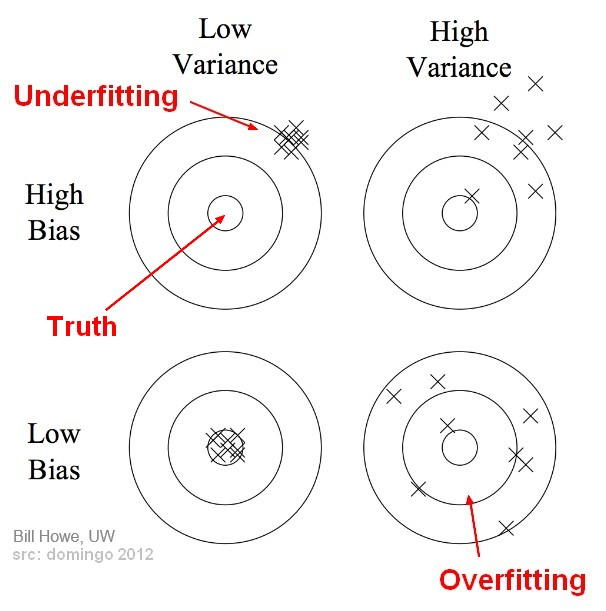Statistics - (Variance|Dispersion|Mean Square) (MS)

The variance shows how widespread the individuals are from the average. The variance is how much that the estimate varies around its average. It's a measure of consistency. A very large variance means...Statistics - Assumptions underlying correlation and regression analysis (Never trust summary statistics alone)

The magnitude of a correlation depends upon many factors, including: Random and representative sampling Measurement of X and Y: Reliability of X and Y Validity of X and Y Several other assumptions:...